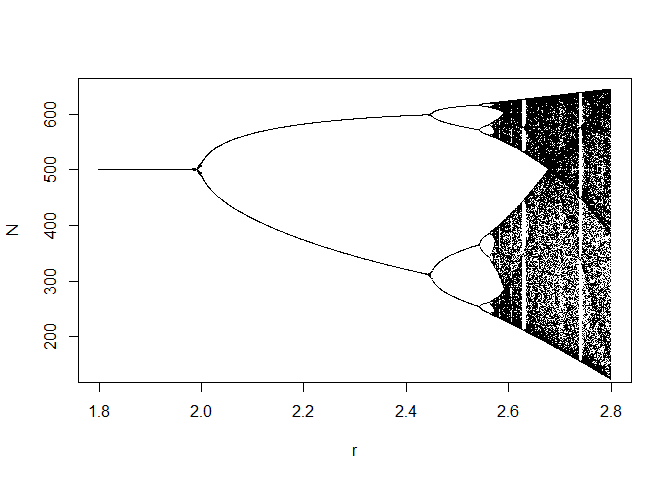# Discrete Logistic Model: Bifurcation Diagram

The Rmd assignment solution file is availabe here.

Simulate $$N_{t+1} = N_t + rN_t(1 - \frac{N_t}{K})$$ using $$K = 500$$, $$N_0 = 100$$, $$r = 1.8, 2.3, 2.45, 2.56, 2.8$$. Compare the results.

K <- 500
r <- c(1.8, 2.3, 2.45, 2.56, 2.8)
t <- 1:100
N <- matrix(nrow = length(t), ncol = length(r))

N[1, ] <- 100
for(i in 1:length(r)) {
for(j in 1:(length(t)-1)) {
N[j+1, i] <- N[j, i] + r[i] * N[j, i] * (1 - (N[j, i] / K))
}
}
colnames(N) <- paste("r =", r)

par(mfrow = c(2, 2))
for(i in 1:ncol(N)) {
plot(t, N[, i], type = "l", ylab = "N", main = colnames(N)[i])
}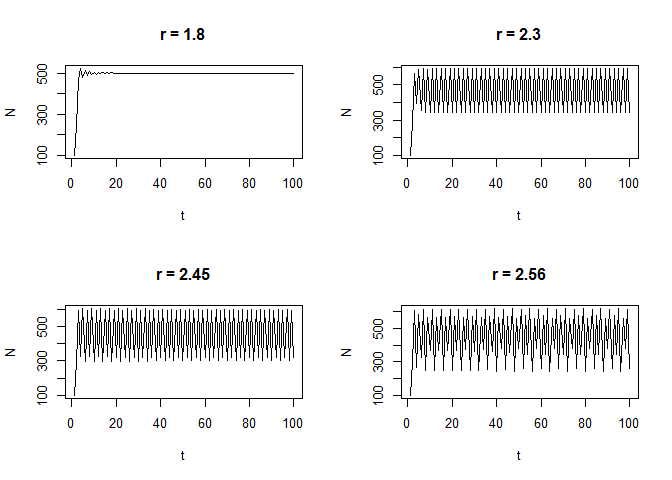par(mfrow = c(1, 1))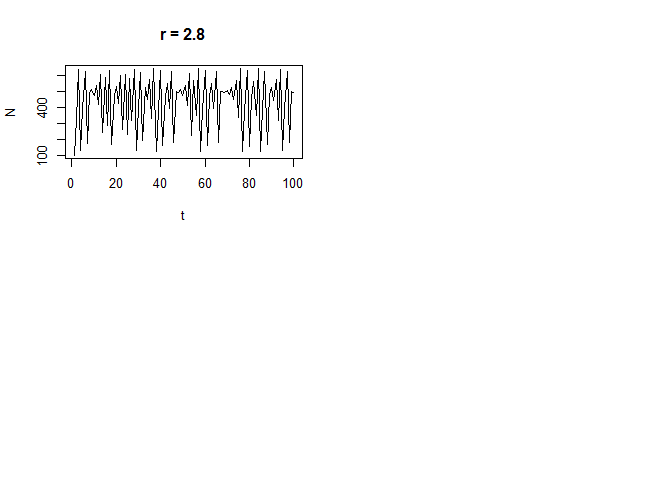Series with r = 1.8, converges to 500, while other series fluctuate around some number and the size of those fluctuations increases as r increases.

Try $$N_0 = 101$$ and compare with Task 1.

M <- matrix(nrow = length(t), ncol = length(r))

M[1, ] <- 101
for(i in 1:length(r)) {
for(j in 1:(length(t)-1)) {
M[j+1, i] <- M[j, i] + r[i] * M[j, i] * (1 - (M[j, i] / K))
}
}
colnames(M) <- paste("r =", r)

par(mfrow = c(2, 2))
for(i in 1:ncol(M)) {
plot(t, M[, i], type = "l", ylab = "M", main = colnames(M)[i])
}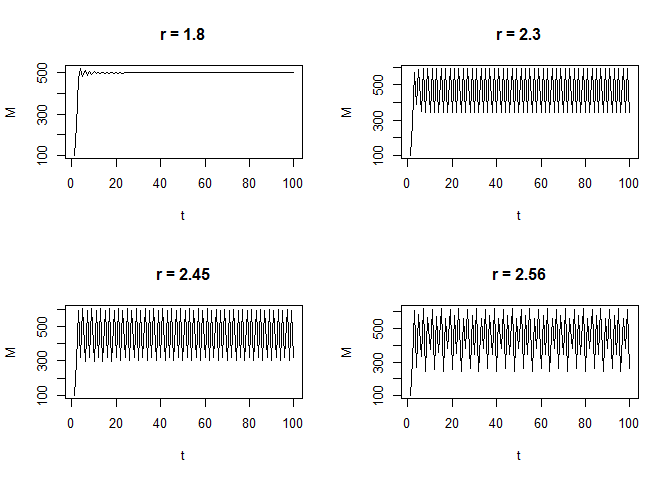par(mfrow = c(1, 1))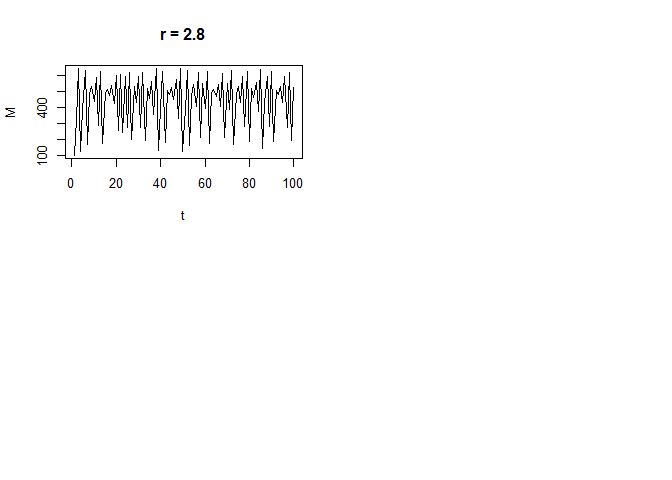Results are very similar compared to Task 1.

Plot time step 1 return map for $$r = 1.8, 2.3, 2.45, 2.56, 2.8$$.

N <- matrix(nrow = length(t), ncol = length(r))

N[1, ] <- 0.0000001
for(i in 1:length(r)) {
for(j in 1:(length(t)-1)) {
N[j+1, i] <- N[j, i] + r[i] * N[j, i] * (1 - (N[j, i] / K))
}
}
colnames(N) <- paste("r =", r)

par(mfrow = c(2, 2))
for(i in 1:ncol(N)) {
plot(N[1:99, i], N[2:100, i], type = "s",
xlab = expression(N[t]), ylab = expression(N[t+1]), main = colnames(N)[i],
xlim = c(0, 1000), ylim = c(0, 1000))
x <- 0:1000
y <- x + r[i] * x * (1 - (x / K))
lines(x, y)
}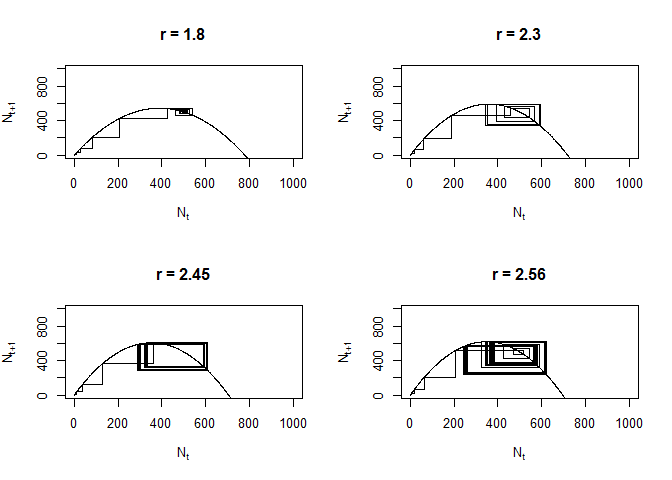par(mfrow = c(1, 1))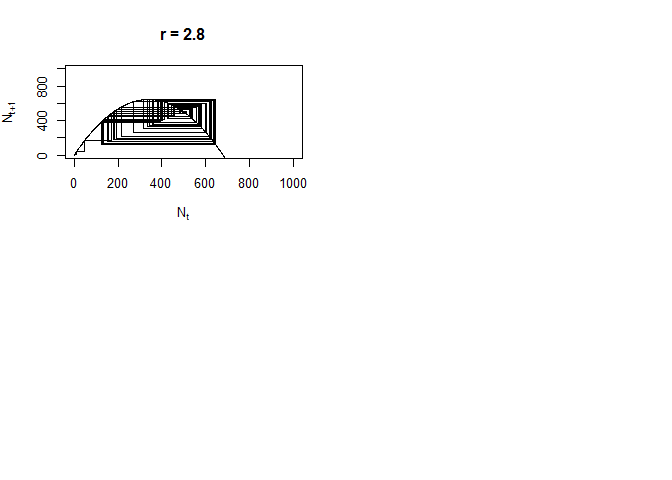Make a bifurcation diagram.

r <- seq(1.8, 2.8, by = 0.001)
t <- 1:200
data <- matrix(nrow = length(t), ncol = length(r))

for(i in 1:length(r)) {
# random initial condition
N <- runif(1, 50, 150)
# first converge to attractor
for(j in t){
N <- N + r[i] * N * (1 - (N / K))
}
# collect points on attractor
for(j in t){
N <- N + r[i] * N * (1 - (N / K))
data[j, i] <- N
}
}

# plot the bifurcation diagram
plot(r, data[1, ], pch = ".", xlab = "r", ylab = "N")
for(i in 2:length(t)) {
points(r, data[i, ], pch = ".")
}